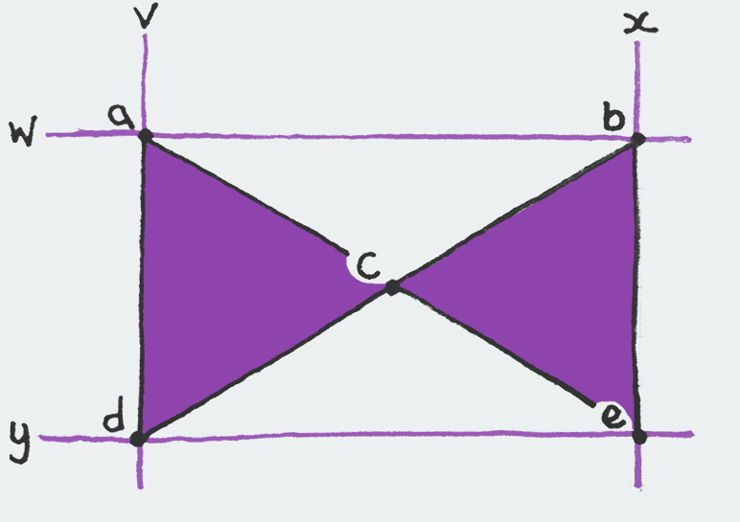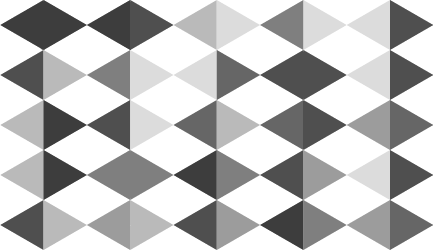Just before Christmas I decided to have a play about with SVG on Codepen. It got quite a lot of attention and I was asked to write a post detailing the process; here is that post.

This idea was born because I needed a way to generate isometric backgrounds on-the-fly with a set colour palette. I decided the best way to do it would be to use a formula that would write some SVG code and apply it to the background of an element. The problem was, I’d never used SVG before and I had no idea what I was doing.

## Polka dots

I decided to start small and get the basics down first. If I could get something simple generated in SVG first then I could move onto writing the formula that would generate my shapes. I created a random polka dot generator that was surprisingly simple.

I've outlined a simplified breakdown of that code below.

``````var width = (dotSize + dotPadding) * dotsWide,
height = (dotSize + dotPadding) * dotsHigh,
background = "<svg xmlns='http://www.w3.org/2000/svg' width='" + width + "' height='" + height + "'>",
color = "#09c",
x,
y;``````

First I define the variables I’m going to use and generate the opening tag for the SVG (assigned to the background variable).

``````for (x = radius; x < width; x += dotSize + dotPadding) {
for (y = radius; y < height; y += dotSize + dotPadding) {
background += "<circle fill='" + color + "' cx='" + x + "' cy='" + y + "' r='" + radius + "'/>";
}
}``````

Then I loop through each circle/dot in the image and append the SVG code to generate it to the background variable.

``background += "</svg>";``

Once each dot has been added, we close the SVG tag.

``````var b64 = 'data:image/svg+xml;base64,' + window.btoa(background),
url = 'url("' + b64 + '")';

\$('html').css('backgroundImage', url);``````

Finally I base64 encode the image before applying it to the background as a Data URI.

## Isometric grid

The polka dot demo proved to me that I could do this. All I had to do now was write the part that generated my isometric triangles. There’s a few ways to do this but I found this way to be the simplest.My plan was to generate this bow-tie shape and fill it with a random colour, chosen from a pre-defined array. I would then repeat this shape so it tessellates. The problem I encountered with this approach is that they didn't stack correctly, and I had with a line of diamond-shaped gaps.

``````for (i = 0; i <= settings.trianglesWide; i += 1) {
for (j = 0; j <= settings.trianglesHigh; j += 1) {
v = i * settings.triangleSize * settings.skew;
w = j * settings.triangleSize;
x = v + (settings.triangleSize * settings.skew);
y = w + settings.triangleSize;
a = v + "," + w;
b = x + "," + w;
c = (v + x) / 2 + "," + ((w + y) / 2);
d = v + "," + y;
e = x + "," + y;

background += "<polygon fill='" + getColor() + "' fill-opacity='" + settings.opacity + "' points='" + a + " " + c + " " + d + " " + "' />";
background += "<polygon fill='" + getColor() + "' fill-opacity='" + settings.opacity + "' points='" + b + " " + c + " " + e + " " + "' />";
}
}``````

Gives you…As you can see, the bow-ties don’t line up quite right but this is fixable. What we need to do is offset every second row by moving it up and along by 50%. I decided to utilise the modulo operation `(j % 1)` to differentiate between even and odd rows. This operation will return a 0 when the number is even and a 1 when it’s odd.

``````for (i = -1; i <= trianglesWide; i += 1) {
for (j = -0.5; j <= trianglesHigh; j += 0.5) {
v = (i + (j % 1)) * triangleSize * skew;
w = j * triangleSize;
x = v + (triangleSize * skew);
y = w + triangleSize;
a = v + "," + w;
b = x + "," + w;
c = (v + x) / 2 + "," + ((w + y) / 2);
d = v + "," + y;
e = x + "," + y;

background += "<polygon fill='" + getColor() + "' fill-opacity='" + opacity + "' points='" + a + " " + c + " " + d + " " + "' />";
background += "<polygon fill='" + getColor() + "' fill-opacity='" + opacity + "' points='" + b + " " + c + " " + e + " " + "' />";
}
}``````

Adding this in allows us to offset the `v` coordinate by 1 on every second row which pushes that row along to the correct point.

You might notice that instead of starting the for loops at 0 I start them at −1 and −0.5 respectively. This is to make sure the pattern starts outside the bounding box so we don’t get jagged edges.

## Colours

Another big part of what I wanted to do here was to use random colours from a selected palette. To do this I wrote a simple `getColor()` function.

``````var colors = ['red', 'blue', 'green'],
getColor = function () {
var hex = colors[Math.floor(Math.random() * colors.length)];
return hex;
};``````

This shouldn’t need too much of an explanation, it returns a random entry from the colours array when it’s called.

## Plugin

Whilst this is not intended to be a jQuery plugin, I wrote it in that manner to allow me to generate several different backgrounds. Have a play about with the settings at the top of the page or check out the demo on Codepen to see just how versatile this can be.